Using Property 5 (Determinant as sum of two or more determinants)

Chapter 4 Class 12 Determinants
Concept wise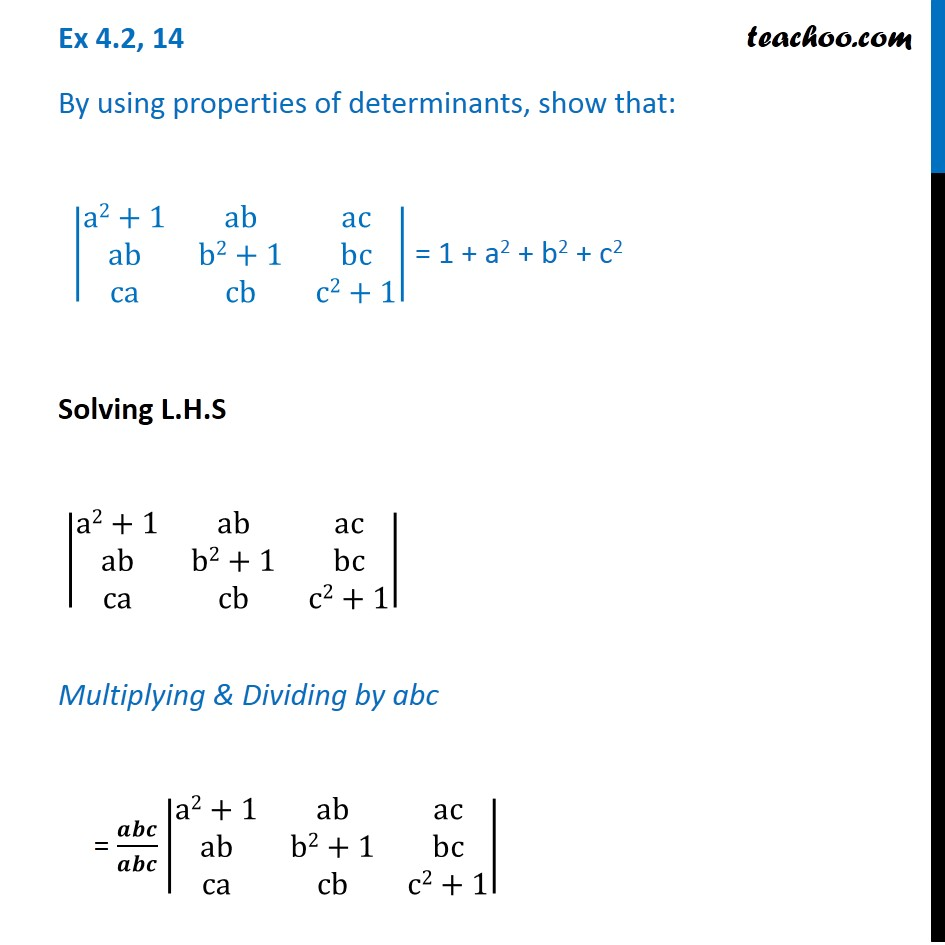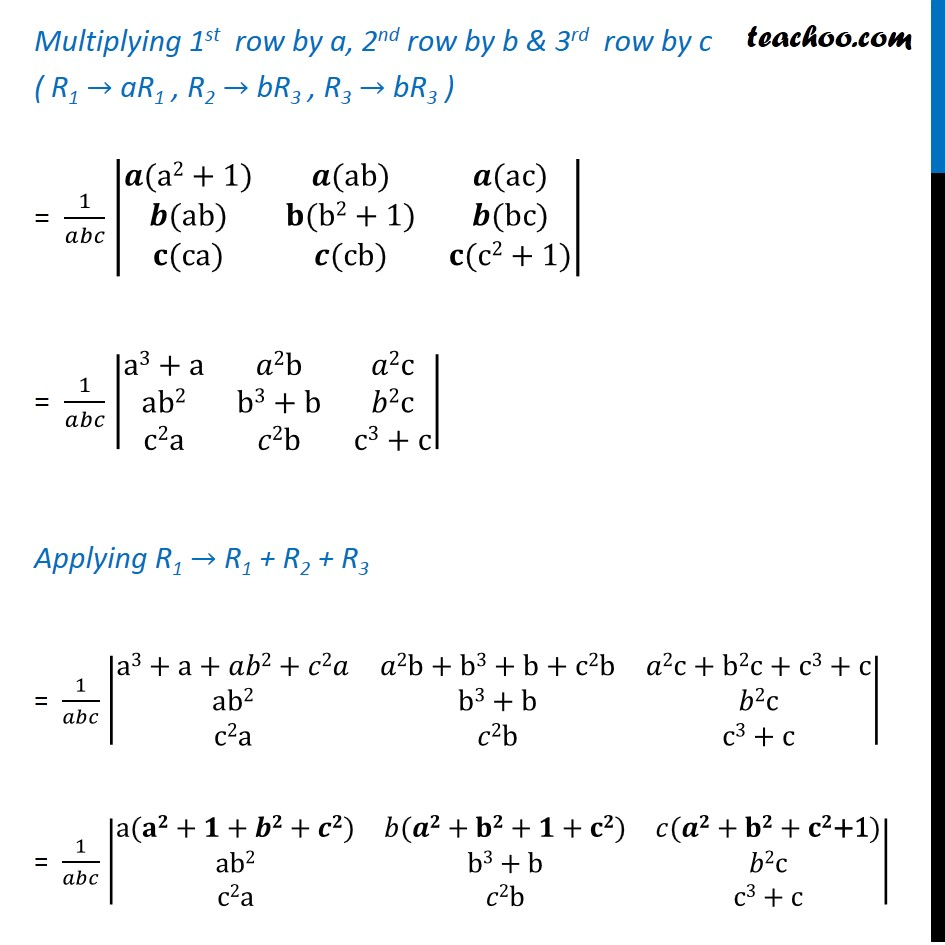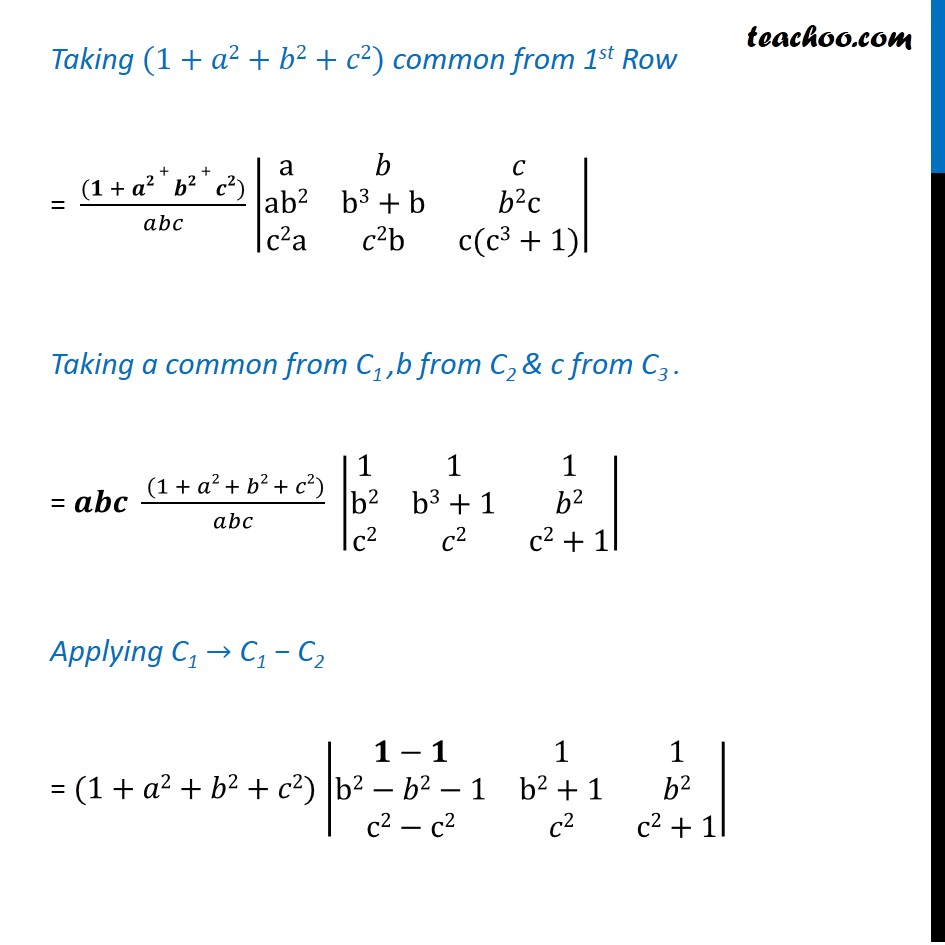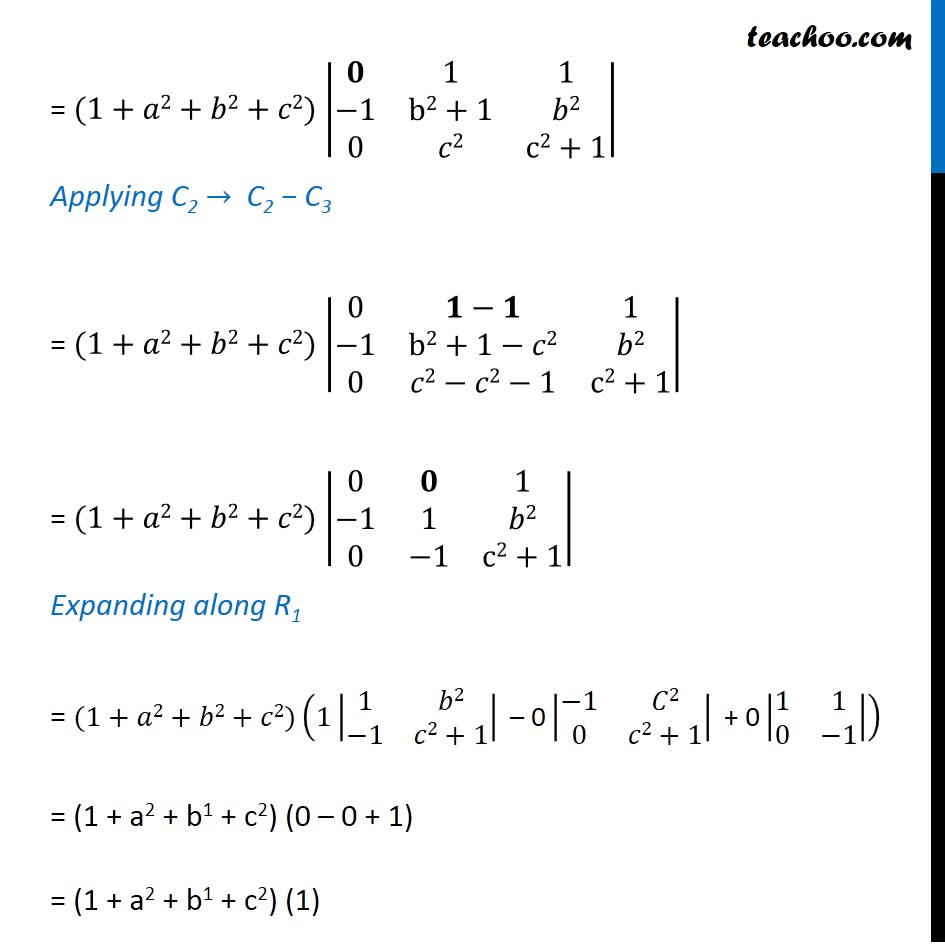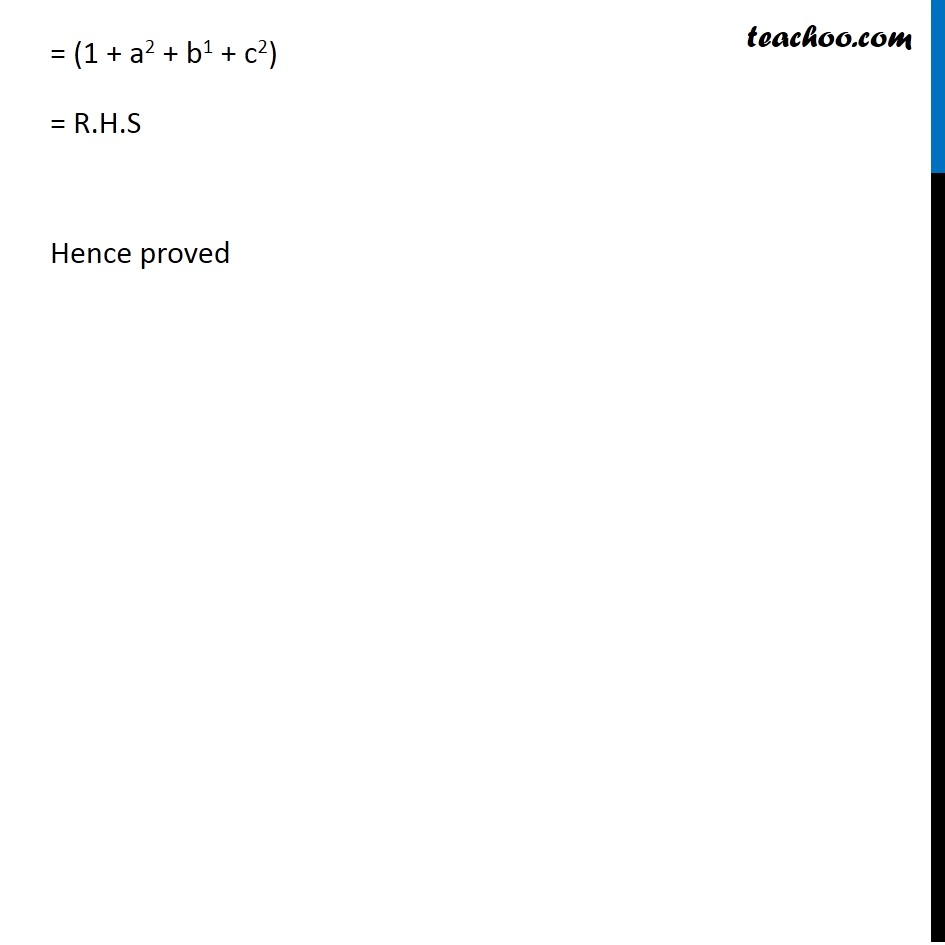Introducing your new favourite teacher - Teachoo Black, at only ₹83 per month

### Transcript

Ex 4.2, 14 By using properties of determinants, show that: |■8(a2+1&ab&ac@ab&b2+1&bc@ca&cb&c2+1)| = 1 + a2 + b2 + c2 Solving L.H.S |■8(a2+1&ab&ac@ab&b2+1&bc@ca&cb&c2+1)| Multiplying & Dividing by abc = 𝒂𝒃𝒄/𝒂𝒃𝒄 |■8(a2+1&ab&ac@ab&b2+1&bc@ca&cb&c2+1)| Multiplying 1st row by a, 2nd row by b & 3rd row by c ( R1 → aR1 , R2 → bR3 , R3 → bR3 ) = 1/𝑎𝑏𝑐 |■8(𝒂(a2+1)&𝒂(ab)&𝒂(ac)@𝒃(ab)&𝐛(b2+1)&𝒃(bc)@𝐜(ca)&𝒄(cb)&𝐜(c2+1))| = 1/𝑎𝑏𝑐 |■8(a3+a&𝑎2b&𝑎2c@ab2&b3+b&𝑏2c@c2a&𝑐2b&c3+c)| Applying R1 → R1 + R2 + R3 = 1/𝑎𝑏𝑐 |■8(a3+a+𝑎𝑏2+𝑐2𝑎&𝑎2b+b3+b+c2b&𝑎2c+b2c+c3+c@ab2&b3+b&𝑏2c@c2a&𝑐2b&c3+c)| = 1/𝑎𝑏𝑐 |■8(a(𝐚𝟐+𝟏+𝒃𝟐+𝒄𝟐)&𝑏(𝒂𝟐+𝐛𝟐+𝟏+𝐜𝟐)&𝑐(𝒂𝟐+𝐛𝟐+𝐜𝟐"+1" )@ab2&b3+b&𝑏2c@c2a&𝑐2b&c3+c)| Taking (1+𝑎2+𝑏2+𝑐2) common from 1st Row = ((𝟏 + 𝒂𝟐 + 𝒃𝟐 + 𝒄𝟐))/𝑎𝑏𝑐 |■8(a&𝑏&𝑐@ab2&b3+b&𝑏2c@c2a&𝑐2b&c(c3+1))| Taking a common from C1 ,b from C2 & c from C3 . = 𝒂𝒃𝒄 ( (1 + 𝑎2 + 𝑏2 + 𝑐2))/𝑎𝑏𝑐 |■8(1&1&1@b2&b3+1&𝑏2@c2&𝑐2&c2+1)| Applying C1 → C1 − C2 = (1+𝑎2+𝑏2+𝑐2) |■8(𝟏−𝟏&1&1@b2−𝑏2−1&b2+1&𝑏2@c2−c2&𝑐2&c2+1)| = (1+𝑎2+𝑏2+𝑐2) |■8(𝟎&1&1@−1&b2+1&𝑏2@0&𝑐2&c2+1)| Applying C2 → C2 − C3 = (1+𝑎2+𝑏2+𝑐2) |■8(0&𝟏−𝟏&1@−1&b2+1−𝑐2&𝑏2@0&𝑐2−𝑐2−1&c2+1)| = (1+𝑎2+𝑏2+𝑐2) |■8(0&𝟎&1@−1&1&𝑏2@0&−1&c2+1)| Expanding along R1 = (1+𝑎2+𝑏2+𝑐2)(1|■8(1&𝑏2@−1&𝑐2+1)|" – 0" |■8(−1&𝐶2@0&𝑐2+1)|" + 0" |■8(1&1@0&−1)|) = (1 + a2 + b1 + c2) (0 – 0 + 1) = (1 + a2 + b1 + c2) (1) = (1 + a2 + b1 + c2) = R.H.S Hence proved